Submitted Successfully!
Thank you for your contribution! You can also upload a video entry or images related to this topic.
Ver. Summary Created by Modification Content Size Created at Operation
1 handwiki -- 5256 2022-10-26 01:33:52

Do you have a full video?

# Confirm

Are you sure to Delete?
Cite
Xu, H. Georg Cantor's First Set Theory Article. Encyclopedia. Available online: https://encyclopedia.pub/entry/32557 (accessed on 02 December 2023).
Xu H. Georg Cantor's First Set Theory Article. Encyclopedia. Available at: https://encyclopedia.pub/entry/32557. Accessed December 02, 2023.
Xu, Handwiki. "Georg Cantor's First Set Theory Article" Encyclopedia, https://encyclopedia.pub/entry/32557 (accessed December 02, 2023).
Xu, H.(2022, November 02). Georg Cantor's First Set Theory Article. In Encyclopedia. https://encyclopedia.pub/entry/32557
Xu, Handwiki. "Georg Cantor's First Set Theory Article." Encyclopedia. Web. 02 November, 2022.
Georg Cantor's First Set Theory Article

Georg Cantor published his first set theory article in 1874, and it contains the first theorems of transfinite set theory, which studies infinite sets and their properties. One of these theorems is "Cantor's revolutionary discovery" that the set of all real numbers is uncountably, rather than countably, infinite. This theorem is proved using Cantor's first uncountability proof, which differs from the more familiar proof using his diagonal argument. The title of the article, "On a Property of the Collection of All Real Algebraic Numbers" ("Ueber eine Eigenschaft des Inbegriffes aller reellen algebraischen Zahlen"), refers to its first theorem: the set of real algebraic numbers is countable. Cantor's article also contains a proof of the existence of transcendental numbers. As early as 1930, mathematicians have disagreed on whether this proof is constructive or non-constructive. Books as recent as 2014 and 2015 indicate that this disagreement has not been resolved. Since Cantor's proof either constructs transcendental numbers or does not, an analysis of his article can determine whether his proof is constructive or non-constructive. Cantor's correspondence with Richard Dedekind shows the development of his ideas and reveals that he had a choice between two proofs, one that uses the uncountability of the real numbers and one that does not. Historians of mathematics have examined Cantor's article and the circumstances in which it was written. For example, they have discovered that Cantor was advised to leave out his uncountability theorem in the article he submitted; he added it during proofreading. They have traced this and other facts about the article to the influence of Karl Weierstrass and Leopold Kronecker. Historians have also studied Dedekind's contributions to the article, including his contributions to the theorem on the countability of the real algebraic numbers. In addition, they have looked at the article's legacy, which includes the impact that the uncountability theorem and the concept of countability have had on mathematics.

transcendental numbers analysis infinite sets

## 1. The Article

Cantor's article is short, less than four and a half pages. It begins with a discussion of the real algebraic numbers and a statement of his first theorem: The set of real algebraic numbers can be put into one-to-one correspondence with the set of positive integers. Cantor restates this theorem in terms more familiar to mathematicians of his time: The set of real algebraic numbers can be written as an infinite sequence in which each number appears only once.

Cantor's second theorem works with a closed interval [ab], which is the set of real numbers ≥ a and ≤ b. The theorem states: Given any sequence of real numbers x1, x2, x3, … and any interval [ab], there is a number in [ab] that is not contained in the given sequence. Hence, there are infinitely many such numbers.

The first part of this theorem implies the "Hence" part. For example, let [0, 1] be the interval, and consider its pairwise disjoint subintervals [0, 1/2], [3/47/8], [15/1631/32], …. Applying the first part of the theorem to each subinterval produces infinitely many numbers in [0, 1] that are not contained in the given sequence.

Cantor observes that combining his two theorems yields a new proof of Liouville's theorem that every interval [ab] contains infinitely many transcendental numbers.

Cantor then remarks that his second theorem is:

the reason why collections of real numbers forming a so-called continuum (such as, all real numbers which are ≥ 0 and ≤ 1) cannot correspond one-to-one with the collection (ν) [the collection of all positive integers]; thus I have found the clear difference between a so-called continuum and a collection like the totality of real algebraic numbers.

This remark contains Cantor's uncountability theorem, which only states that an interval [ab] cannot be put into one-to-one correspondence with the set of positive integers. It does not state that this interval is an infinite set of larger cardinality than the set of positive integers. Cardinality is defined in Cantor's next article, which was published in 1878.

Cantor only states his uncountability theorem. He does not use it in any proofs.

## 2. The Proofs

### 2.1. First Theorem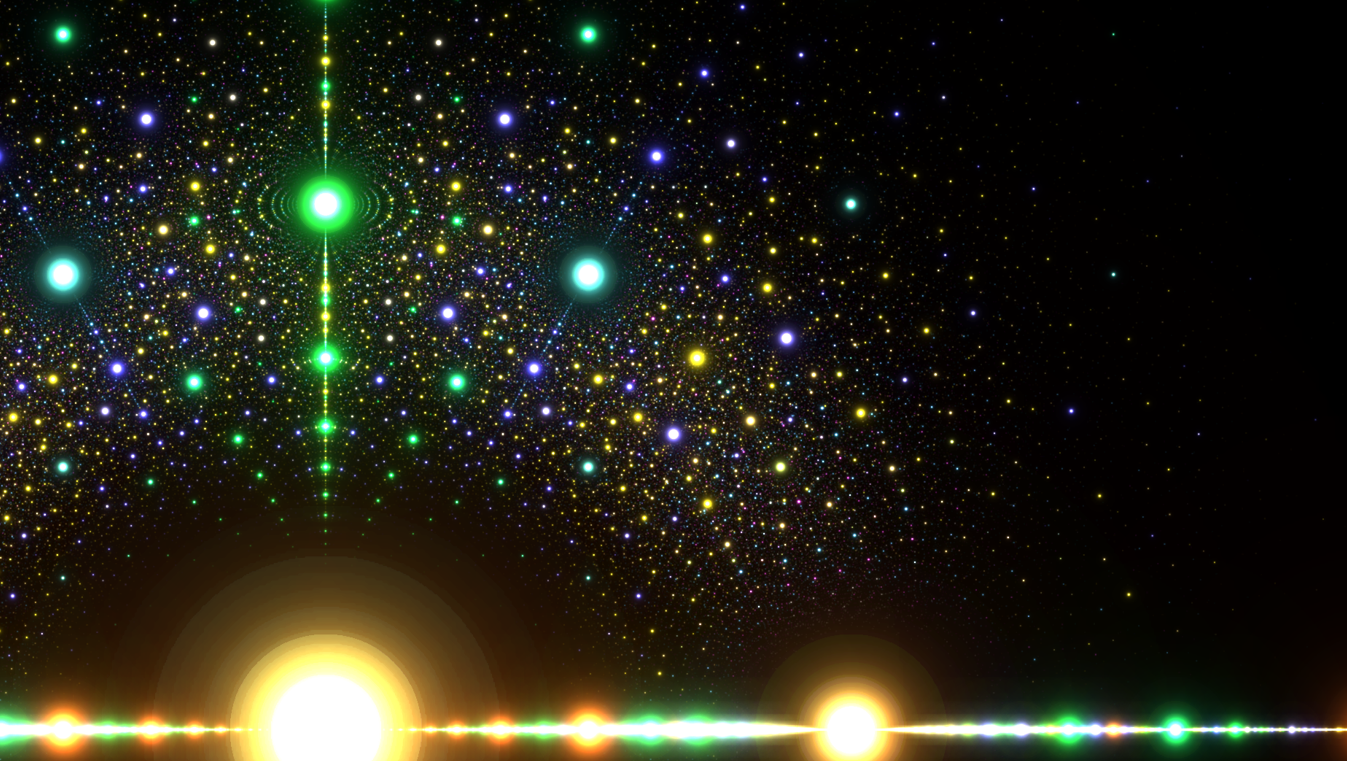Algebraic numbers on the complex plane colored by polynomial degree. (red = 1, green = 2, blue = 3, yellow = 4). Points become smaller as the integer polynomial coefficients become larger.

To prove that the set of real algebraic numbers is countable, define the height of a polynomial of degree n with integer coefficients as: n − 1 + |a0| + |a1| + … + |an|, where a0, a1, …, an are the coefficients of the polynomial. Order the polynomials by their height, and order the real roots of polynomials of the same height by numeric order. Since there are only a finite number of roots of polynomials of a given height, these orderings put the real algebraic numbers into a sequence. Cantor went a step further and produced a sequence in which each real algebraic number appears just once. He did this by only using polynomials that are irreducible over the integers. The table below contains the beginning of Cantor's enumeration.

### 2.2. Second Theorem

Only the first part of Cantor's second theorem needs to be proved. It states: Given any sequence of real numbers x1, x2, x3, … and any interval [ab], there is a number in [ab] that is not contained in the given sequence. We simplify Cantor's proof by using open intervals. The open interval (ab) is the set of real numbers > a and < b.

To find a number in [ab] that is not contained in the given sequence, construct two sequences of real numbers as follows: Find the first two numbers of the given sequence that are in (ab). Denote the smaller of these two numbers by a1 and the larger by b1. Similarly, find the first two numbers of the given sequence that are in (a1b1). Denote the smaller by a2 and the larger by b2. Continuing this procedure generates a sequence of intervals (a1b1), (a2b2), (a3b3), … such that each interval in the sequence contains all succeeding intervals—that is, it generates a sequence of nested intervals. This implies that the sequence a1, a2, a3, … is increasing and the sequence b1, b2, b3, … is decreasing.

Either the number of intervals generated is finite or infinite. If finite, let (aNbN) be the last interval. If infinite, take the limits a = limn → ∞ an and b = limn → ∞ bn. Since an < bn for all n, either a = b or a < b. Thus, there are three cases to consider: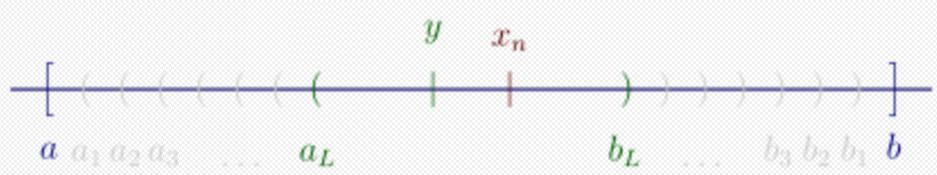Case 1: Last interval (aN, bN)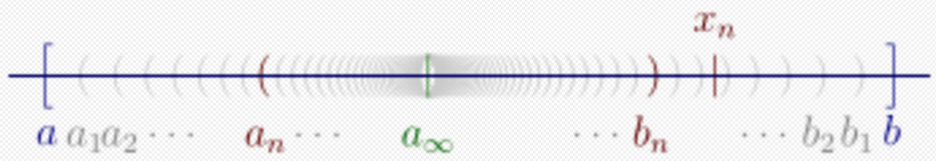Case 2: a = b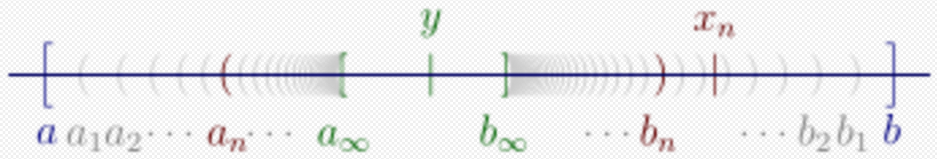Case 3: a < b
• Case 1: There is a last interval (aNbN). Since at most one xn can be in this interval, every y in this interval except xn (if it exists) is not contained in the given sequence.
• Case 2: a = b. Then a is not contained in the given sequence since for all n: a belongs to the interval (anbn), but as Cantor observes, xn does not. This observation is implied by the following proof.
• Case 3: a < b. Then every y in [ab] is not contained in the given sequence since for all n: y belongs to (anbn) but xn does not.

The proof is complete since, in all cases, at least one real number in [ab] has been found that is not contained in the given sequence.

Cantor's proofs are constructive and have been used to write a computer program that generates the digits of a transcendental number. This program applies Cantor's construction to a sequence containing all the real algebraic numbers between 0 and 1. The article that discusses this program gives some of its output, which shows how the construction generates a transcendental.

### 2.3. Dense Sequences

Cantor observes that a = b when his construction is applied to the sequence of real algebraic numbers. This sequence is dense in [ab], which means that every open subinterval (yz) of [ab] contains a term of the sequence. Cantor's observation follows from the lemma: If the sequence is dense in [ab], then a = b.

We prove the contrapositive: If a ≠ b, then the sequence is not dense in [ab]. If a ≠ b, then case 2 does not occur. Hence, there are two cases to consider:

• Case 1: There is a last interval (aNbN), so at most one xn can be in this interval. If there is such an xn, then the interval (aNxn) contains no terms of the sequence. Otherwise, the interval (aNbN) contains no terms of the sequence.
• Case 3: a < b. The interval (ab) contains no terms of the sequence.

In both cases, an open subinterval of [ab] has been found that contains no terms of the sequence, which implies that the sequence is not dense in [ab]. Therefore, the contrapositive of the lemma is true, which implies that the lemma is true.

### 2.4. Example of Cantor's Construction

An example illustrates how Cantor's construction works. Consider the sequence: 1/2, 1/3, 2/3, 1/4, 3/4, 1/5, 2/5, 3/5, 4/5, … This sequence is obtained by ordering the rational numbers in (0, 1) by increasing denominators, ordering those with the same denominator by increasing numerators, and omitting reducible fractions. Since this sequence is dense in [0, 1], the construction generates infinitely many intervals. The table below shows the first five steps of the construction. The table's first column contains the intervals (anbn). The second column lists the terms visited during the search for the first two terms in (anbn). These two terms are in red.

Generating a number using Cantor's construction
Interval Finding the next interval Interval (decimal)
$\displaystyle{ \left(\;\frac{1}{3}, \;\;\!\frac{1}{2}\;\right) }$ $\displaystyle{ \;\frac{2}{3}, \;\! \frac{1}{4}, \;\! \frac{3}{4}, \;\! \frac{1}{5}, \;\! {\color{red}\frac{2}{5},}\;\! \;\! \frac{3}{5}, \;\! \frac{4}{5}, \;\! \frac{1}{6}, \;\! \frac{5}{6}, \;\! \frac{1}{7}, \;\! \frac{2}{7}, \;\! {\color{red}\frac{3}{7}} }$ $\displaystyle{ \left(0.3333\dots,\; 0.5000\dots\right) }$
$\displaystyle{ \left(\;\frac{2}{5}, \;\;\!\frac{3}{7}\;\right) }$ $\displaystyle{ \;\frac{4}{7}, \;\dots,\; \frac{1}{12}, {\color{red}\frac{5}{12},}\;\! \frac{7}{12}, \;\dots,\; \frac{6}{17}, {\color{red}\frac{7}{17}} }$ $\displaystyle{ \left(0.4000\dots,\; 0.4285\dots\right) }$
$\displaystyle{ \left(\frac{7}{17}, \frac{5}{12}\right) }$ $\displaystyle{ \frac{8}{17}, \;\!\dots,\;\! \frac{11}{29}, {\color{red}\frac{12}{29},}\;\! \frac{13}{29}, \;\dots,\; \frac{16}{41}, {\color{red}\frac{17}{41}} }$ $\displaystyle{ \left(0.4117\dots,\; 0.4166\dots\right) }$
$\displaystyle{ \left(\frac{12}{29}, \frac{17}{41}\right) }$ $\displaystyle{ \frac{18}{41}, \;\!\dots,\;\! \frac{27}{70}, {\color{red}\frac{29}{70},}\;\! \frac{31}{70}, \;\dots,\; \frac{40}{99}, {\color{red}\frac{41}{99}} }$ $\displaystyle{ \left(0.4137\dots,\; 0.4146\dots\right) }$
$\displaystyle{ \left(\frac{41}{99}, \frac{29}{70}\right) }$ $\displaystyle{ \frac{43}{99}, \dots, \frac{69}{169}, {\color{red}\frac{70}{169},}\;\! \frac{71}{169}, \,\dots, \frac{98}{239}, {\color{red}\frac{99}{239}} }$ $\displaystyle{ \left(0.4141\dots,\; 0.4142\dots\right) }$

Since the sequence contains all the rational numbers in (0, 1), the construction generates an irrational number, which turns out to be √2 − 1.

## 3. The Development of Cantor's Ideas

The development leading to Cantor's article appears in the correspondence between Cantor and Richard Dedekind. On November 29, 1873, Cantor asked Dedekind whether the collection of positive integers and the collection of positive real numbers "can be corresponded so that each individual of one collection corresponds to one and only one individual of the other?" Cantor added that collections having such a correspondence include the collection of positive rational numbers, and collections of the form (an1n2, . . . , nν) where n1, n2, . . . , nν, and ν are positive integers.

Dedekind replied that he was unable to answer Cantor's question, and said that it "did not deserve too much effort because it has no particular practical interest." Dedekind also sent Cantor a proof that the set of algebraic numbers is countable.

On December 2, Cantor responded that his question does have interest: "It would be nice if it could be answered; for example, provided that it could be answered no, one would have a new proof of Liouville's theorem that there are transcendental numbers."

On December 7, Cantor sent Dedekind a proof by contradiction that the set of real numbers is uncountable. Cantor starts by assuming the real numbers can be written as a sequence. Then he applies a construction to this sequence to produce a real number not in the sequence, thus contradicting his assumption. The letters of December 2 and 7 lead to a non-constructive proof of the existence of transcendental numbers.

On December 9, Cantor announced the theorem that allowed him to construct transcendental numbers as well as prove the uncountability of the set of real numbers:

(I)     ω1, ω2, … , ωn, …
I can determine, in every given interval [α, β], a number η that is not included in (I).

This is the second theorem in Cantor's article. It comes from realizing that his construction can be applied to any sequence, not just to sequences that supposedly enumerate the real numbers. So Cantor had a choice between two proofs that demonstrate the existence of transcendental numbers: one proof is constructive, but the other is not. We now compare the proofs assuming that we have a sequence consisting of all the real algebraic numbers.

The constructive proof applies Cantor's construction to this sequence and the interval [ab] to produce a transcendental number in this interval.

The non-constructive proof uses two proofs by contradiction:

1. The proof by contradiction used to prove the uncountability theorem (see Proof of Cantor's uncountability theorem).
2. The proof by contradiction used to prove the existence of transcendental numbers from the countability of the real algebraic numbers and the uncountability of real numbers. Cantor's December 2nd letter mentions this existence proof but does not contain it. Here is a proof: Assume that there are no transcendental numbers in [ab]. Then all the numbers in [ab] are algebraic. This implies that they form a subsequence of the sequence of all real algebraic numbers, which contradicts Cantor's uncountability theorem. Thus, the assumption that there are no transcendental numbers in [ab] is false. Therefore, there is a transcendental number in [ab].

Cantor chose to publish the constructive proof, which not only produces a transcendental number but is also shorter and avoids two proofs by contradiction.

The non-constructive proof from Cantor's correspondence is simpler than the one above because it works with all the real numbers rather than the interval [ab]. This eliminates the subsequence step and all occurrences of [ab] in the second proof by contradiction.

## 4. The Disagreement about Cantor's Existence Proof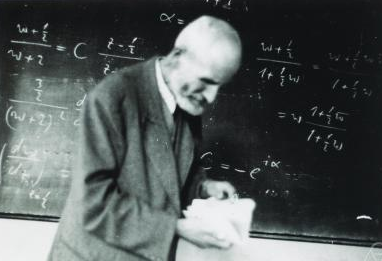Oskar Perron

The correspondence containing Cantor's non-constructive reasoning was published in 1937. By then, other mathematicians had rediscovered its non-constructive proof. As early as 1921, this proof was attributed to Cantor and criticized for not producing any transcendental numbers. In that year, Oskar Perron stated: "… Cantor's proof for the existence of transcendental numbers has, along with its simplicity and elegance, the great disadvantage that it is only an existence proof; it does not enable us to actually specify even a single transcendental number."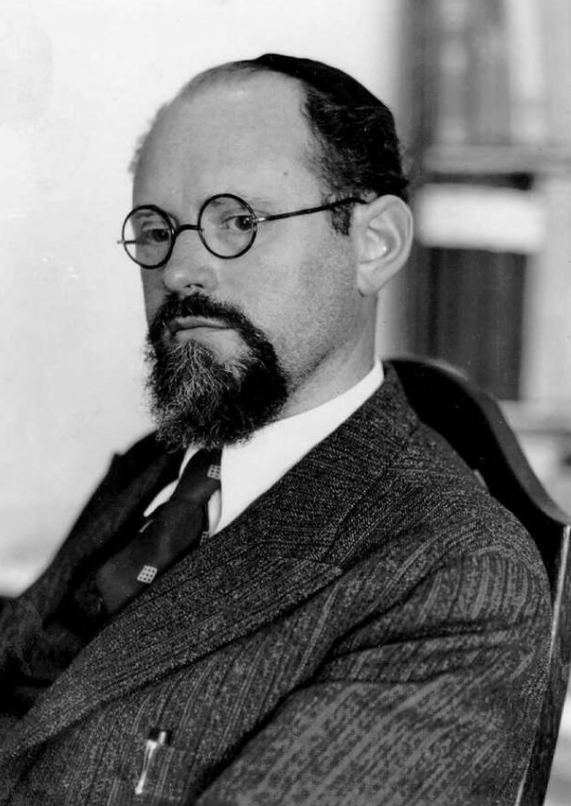Abraham Fraenkel

Some mathematicians have attempted to correct this misunderstanding of Cantor's work. In 1930, the set theorist Abraham Fraenkel stated that Cantor's method is "… a method that incidentally, contrary to a widespread interpretation, is fundamentally constructive and not merely existential." In 1972, Irving Kaplansky wrote: "It is often said that Cantor's proof is not 'constructive,' and so does not yield a tangible transcendental number. This remark is not justified. If we set up a definite listing of all algebraic numbers … and then apply the diagonal procedure …, we get a perfectly definite transcendental number (it could be computed to any number of decimal places)."

Cantor's diagonal argument has often replaced his 1874 construction in expositions of his proof. The diagonal argument is constructive and produces a more efficient computer program than his 1874 construction. Using it, a computer program has been written that computes the digits of a transcendental number in polynomial time. The program that uses Cantor's 1874 construction requires at least sub-exponential time.

The disagreement about Cantor's proof occurs because two groups of mathematicians are talking about different proofs: the constructive one that Cantor published and the non-constructive one that was later rediscovered. The opinion that Cantor's proof is non-constructive appears in some books that were quite successful as measured by the length of time new editions or reprints appeared—for example: Eric Temple Bell's Men of Mathematics (1937; still being reprinted), Godfrey Hardy and E. M. Wright's An Introduction to the Theory of Numbers (1938; 2008 6th edition), Garrett Birkhoff and Saunders Mac Lane's A Survey of Modern Algebra (1941; 1997 5th edition), and Michael Spivak's Calculus (1967; 2008 4th edition). Since these books view Cantor's proof as non-constructive, they do not mention his constructive proof. On the other hand, the quotations above from Fraenkel and Kaplansky show that they knew Cantor's work can be used non-constructively. The disagreement about Cantor's proof shows no sign of being resolved: since 2014, at least two books have appeared stating that Cantor's proof is constructive, and at least four have appeared stating that his proof does not construct any (or a single) transcendental.

Asserting that Cantor gave a non-constructive proof can lead to erroneous statements about the history of mathematics. In A Survey of Modern Algebra, Birkhoff and Mac Lane state: "Cantor's argument for this result [Not every real number is algebraic] was at first rejected by many mathematicians, since it did not exhibit any specific transcendental number." Birkhoff and Mac Lane are talking about the non-constructive proof. Cantor's proof produces transcendental numbers, and there appears to be no evidence that his argument was rejected. Even Leopold Kronecker, who had strict views on what is acceptable in mathematics and who could have delayed publication of Cantor's article, did not delay it. In fact, applying Cantor's construction to the sequence of real algebraic numbers produces a limiting process that Kronecker accepted—namely, it determines a number to any required degree of accuracy.

## 5. The Influence of Weierstrass and Kronecker on Cantor's Article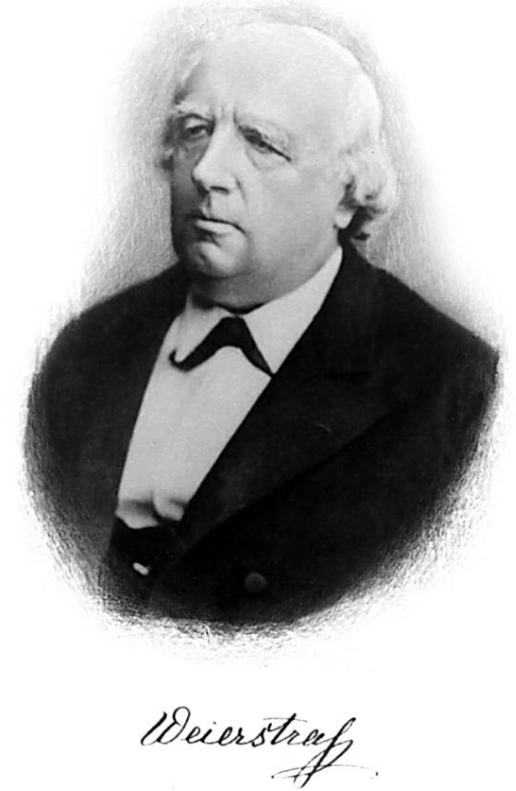Karl Weierstrass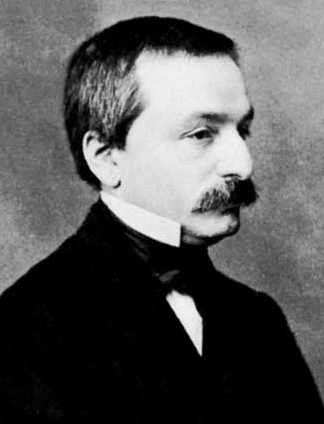Leopold Kronecker, 1865

Historians of mathematics have discovered the following facts about Cantor's article "On a Property of the Collection of All Real Algebraic Numbers":

• Cantor's uncountability theorem was left out of the article he submitted. He added it during proofreading.
• The article's title refers to the set of real algebraic numbers. The main topic in Cantor's correspondence was the set of real numbers.
• The proof of Cantor's second theorem came from Dedekind. However, it omits Dedekind's explanation of why the limits a and b exist.
• Cantor restricted his first theorem to the set of real algebraic numbers. The proof he was using demonstrates the countability of the set of all algebraic numbers.

To explain these facts, historians have pointed to the influence of Cantor's former professors, Karl Weierstrass and Leopold Kronecker. Cantor discussed his results with Weierstrass on December 23, 1873. Weierstrass was first amazed by the concept of countability, but then found the countability of the set of real algebraic numbers useful. Cantor did not want to publish yet, but Weierstrass felt that he must publish at least his results concerning the algebraic numbers.

From his correspondence, it appears that Cantor only discussed his article with Weierstrass. However, Cantor told Dedekind: "The restriction which I have imposed on the published version of my investigations is caused in part by local circumstances …" Cantor biographer Joseph Dauben believes that "local circumstances" refers to Kronecker who, as a member of the editorial board of Crelle's Journal, had delayed publication of an 1870 article by Eduard Heine, one of Cantor's colleagues. Cantor would submit his article to Crelle's Journal.

Weierstrass advised Cantor to leave his uncountability theorem out of the article he submitted, but Weierstrass also told Cantor that he could add it as a marginal note during proofreading, which he did. It appears in a remark at the end of the article's introduction. The opinions of Kronecker and Weierstrass both played a role here. Kronecker did not accept infinite sets, and it seems that Weierstrass did not accept that two infinite sets could be so different, with one being countable and the other not. Weierstrass changed his opinion later. Without the uncountability theorem, the article needed a title that did not refer to this theorem. Cantor chose Ueber eine Eigenschaft des Inbegriffes aller reellen algebraischen Zahlen ("On a Property of the Collection of All Real Algebraic Numbers"), which refers to the countability of the set of real algebraic numbers, the result that Weierstrass found useful.

Kronecker's influence appears in the proof of Cantor's second theorem. Cantor used Dedekind's version of the proof except he left out why the limits a = limn → ∞ an and b = limn → ∞ bn exist. Dedekind had used his "principle of continuity" to prove they exist. This principle (which is equivalent to the least upper bound property of the real numbers) comes from Dedekind's construction of the real numbers, a construction Kronecker did not accept.

Cantor restricted his first theorem to the set of real algebraic numbers even though Dedekind had sent him a proof that handled all algebraic numbers. Cantor did this for expository reasons and because of "local circumstances." This restriction simplifies the article because the second theorem works with real sequences. Hence, the construction in the second theorem can be applied directly to the enumeration of the real algebraic numbers to produce "an effective procedure for the calculation of transcendental numbers." This procedure would be acceptable to Weierstrass.

## 6. Dedekind's Contributions to Cantor's ArticleRichard Dedekind, c. 1870.

Since 1856, Dedekind had developed theories involving infinitely many infinite sets—for example: ideals, which he used in algebraic number theory, and Dedekind cuts, which he used to construct the real numbers. This work enabled him to understand and contribute to Cantor's work.

Dedekind's first contribution concerns the theorem that the set of real algebraic numbers is countable. Cantor is usually given credit for this theorem, but the mathematical historian José Ferreirós calls it "Dedekind's theorem." Their correspondence reveals what each mathematician contributed to the theorem.

In his letter introducing the concept of countability, Cantor stated without proof that the set of positive rational numbers is countable, as are sets of the form (an1n2, …, nν) where n1n2, …, nν, and ν are positive integers. Cantor's second result uses indexed numbers: a set of the form (an1n2, …, nν) is the range of a function from the ν indices to the set of real numbers. His second result implies his first: let ν = 2 and an1n2 = n1/n2. The function can be quite general—for example, an1n2n3n4n5 = (n1/n2)1/n3 + tan(n4/n5).

Dedekind replied with a proof of the theorem that the set of all algebraic numbers is countable. To obtain this result from Cantor's theorem about indexed numbers, Dedekind had to remove the restriction to positive integer indices and realize that the ordering produced can order the polynomials that have integer coefficients.

In his reply, Cantor did not claim to have proved Dedekind's result. He did indicate how he proved his theorem about indexed numbers: "Your proof that (n) [the set of positive integers] can be correlated one-to-one with the field of all algebraic numbers is approximately the same as the way I prove my contention in the last letter. I take n12 + n22 + ··· + nν2 = $\displaystyle{ \mathfrak{N} }$ and order the elements accordingly." Cantor's ordering cannot handle indices that are 0.

Dedekind's second contribution is his proof of Cantor's second theorem. Dedekind sent Cantor this proof in reply to Cantor's letter that announced the uncountability theorem and proved it using infinitely many sequences. Before Dedekind's proof arrived, Cantor wrote that he had found a simpler proof that did not use infinitely many sequences. So Cantor had a choice of proofs and chose to publish Dedekind's.

Cantor thanked Dedekind privately for his help: "… your comments (which I value highly) and your manner of putting some of the points were of great assistance to me." However, he did not mention Dedekind's help in his article. In previous articles, he had acknowledged help received from Kronecker, Weierstrass, Heine, and Hermann Schwarz. Cantor's failure to mention Dedekind's contributions damaged his relationship with Dedekind. Dedekind stopped replying to his letters and did not resume the correspondence until October 1876.

## 7. The Legacy of Cantor's Article

Cantor's article introduced the uncountability theorem and the concept of countability. Both would lead to significant developments in mathematics.

The uncountability theorem demonstrated that one-to-one correspondences can be used to analyze infinite sets. In 1878, Cantor used them to define and compare cardinalities. He also constructed one-to-one correspondences to prove that the n-dimensional spaces Rn (where R is the set of real numbers) and the set of irrational numbers have the same cardinality as R.

In 1883, Cantor extended the natural numbers with his infinite ordinals. This extension was necessary for his work on the Cantor–Bendixson theorem. Cantor discovered other uses for the ordinals—for example, he used sets of ordinals to produce an infinity of sets having different infinite cardinalities. His work on infinite sets together with Dedekind's set-theoretical work created set theory.

The concept of countability led to countable operations and objects that are used in various areas of mathematics. For example, in 1878, Cantor introduced countable unions of sets. In the 1890s, Émile Borel used countable unions in his theory of measure, and René Baire used countable ordinals to define his classes of functions. Building on the work of Borel and Baire, Henri Lebesgue created his theories of measure and integration, which were published from 1899 to 1901.

Countable models are used in set theory. In 1922, Thoralf Skolem proved that if conventional axioms of set theory are consistent, then they have a countable model. Since this model is countable, its set of real numbers is countable. This consequence is called Skolem's paradox, and Skolem explained why it does not contradict Cantor's uncountability theorem: although there is a one-to-one correspondence between this set and the set of positive integers, no such one-to-one correspondence is a member of the model. Thus the model considers its set of real numbers to be uncountable, or more precisely, the first-order sentence that says the set of real numbers is uncountable is true within the model. In 1963, Paul Cohen used countable models to prove his independence theorems.

### References

1. In letter to Dedekind dated December 25, 1873, Cantor states that he has written and submitted "a short paper" titled On a Property of the Collection of All Real Algebraic Numbers. Noether & Cavaillès 1937, p. 17; English translation: Ewald 1996, p. 847.
2. Cantor 1874. English translation: Ewald 1996, pp. 840–843.
3. Gray 1994, p. 828.
4. Cantor 1874, p. 259. English translation: Ewald 1996, pp. 840–841.
5. Cantor 1874, p. 259. English translation: Gray 1994, p. 820.
6. Cantor 1878, p. 242.
7. Our proof is nearly the same as the proof of Corollary 2 in Gray 1994, p. 820. The only difference is that we specify the contradiction.
8. Cantor 1874, pp. 259–260. English translation: Ewald 1996, p. 841.
9. Cantor 1874, pp. 260–261. English translation: Ewald 1996, pp. 841–842.
10. Cantor 1874, p. 261. English translation: Ewald 1996, p. 842.
11. The difference between our proof and Cantor's is that he generates the sequence of closed intervals [an, bn]. To find an + 1 and bn + 1, he must also use the open intervals (an, bn). By generating a sequence of open intervals, we avoid working with the closed intervals.
12. Gray 1994, p. 822.
13. This example is nearly the same as an exercise in Gray 1994, p. 823. The only difference is that this sequence contains only irreducible fractions, while Gray's sequence includes the reducible fractions of the initial interval.
14. LeVeque 1956, pp. 154–155.
15. LeVeque 1956, p. 174.
16. Weisstein 2003, p. 541.
17. Noether & Cavaillès 1937, pp. 12–13. English translation: Gray 1994, p. 827; Ewald 1996, p. 844.
18. Noether & Cavaillès 1937, p. 18. English translation: Ewald 1996, p. 848.
19. Noether & Cavaillès 1937, p. 13. English translation: Gray 1994, p. 827.
20. Noether & Cavaillès 1937, pp. 14–15. English translation: Ewald 1996, pp. 845–846.
21. Noether & Cavaillès 1937, p. 16. English translation: Gray 1994, p. 827.
22. The beginning of our proof is derived from the proof below by restricting the numbers in this proof to the interval [a, b]. However, we derive the contradiction by using a subsequence because Cantor was using sequences in his 1873 work on countability. Satz 68. Es gibt transzendente Zahlen.Gäbe es nämlich keine transzendenten Zahlen, so wären alle Zahlen algebraisch, das Kontinuum also identisch mit der Menge aller algebraischen Zahlen. Das ist aber unmöglich, weil die Menge aller algebraischen Zahlen abzählbar ist, das Kontinuum aber nicht.Translation: Theorem 68. There are transcendental numbers. If there were no transcendental numbers, then all numbers would be algebraic. Hence, the continuum would be identical to the set of all algebraic numbers. However, this is impossible because the set of all algebraic numbers is countable, but the continuum is not.
23. Gray 1994, pp. 827–828.
24. Perron 1921, p. 162. English translation: Gray 1994, p. 828.
25. Fraenkel 1930, p. 237. English translation: Gray 1994, p. 823.
26. Kaplansky 1972, p. 25.
27. The program using the diagonal method produces $\displaystyle{ n }$ digits in $\displaystyle{ {\colorO}(n^2 \log^2 n \log \log n) }$ steps, while the program using the 1874 method requires at least $\displaystyle{ O(2^{\sqrt}) }$ steps to produce $\displaystyle{ n }$ digits. (Gray 1994, pp. 822–823.)
28. Bell 1937, pp. 568–569; Hardy & Wright 1938, p. 159 (6th ed., pp. 205–206); Birkhoff & Mac Lane 1941, p. 392, (5th ed., pp. 436–437); Spivak 1967, pp. 369–370 (4th ed., pp. 448–449).
29. Proof is constructive: Dasgupta 2014, p. 107; Sheppard 2014, pp. 131–132. Proof is non-constructive: Jarvis 2014, p. 18; Chowdhary 2015, p. 19; Stewart 2015, p. 285; Stewart & Tall 2015, p. 333.
30. Birkhoff & Mac Lane 1941, p. 392, (5th ed., pp. 436–437).
31. Gray 1994, p. 828.
32. Edwards 1989; Gray 1994, p. 828.
33. Edwards 1989, pp. 74–75.
34. Kronecker's opinion was: "Definitions must contain the means of reaching a decision in a finite number of steps, and existence proofs must be conducted so that the quantity in question can be calculated with any required degree of accuracy." So Kronecker would accept Cantor's argument as a valid existence proof, but he would not accept its conclusion that transcendental numbers exist. For Kronecker, they do not exist because their definition contains no means for deciding in a finite number of steps whether or not a given number is transcendental. To prove that Cantor's construction calculates numbers to any required degree of accuracy, we need to prove: Given a k, an n can be computed such that bn – an ≤ 1/k where (an, bn) is the n-th interval of Cantor's construction. An example of how to prove this is given in Gray 1994, p. 822. Cantor's diagonal argument provides an accuracy of 10−n after n real algebraic numbers have been calculated because each of these numbers generates one digit of the transcendental number.
35. Ferreirós 2007, p. 184.
36. Noether & Cavaillès 1937, pp. 12–16. English translation: Ewald 1996, pp. 843–846.
37. Dauben 1979, p. 67.
38. Noether & Cavaillès 1937, pp. 16–17. English translation: Ewald 1996, p. 847.
39. Grattan-Guinness 1971, p. 124.
40. Dauben 1979, pp. 67, 308–309.
41. See "The article" section. Also: Cantor 1874, p. 259; English translation: Ewald 1996, p. 841.
42. Ferreirós 2007, pp. 184–185, 245.
43. "It is unclear when his attitude changed, but there is evidence that by the mid-1880s he was accepting the conclusion that infinite sets are of different powers [cardinalities]." (Ferreirós 2007)
44. Ferreirós 2007, p. 177.
45. Dauben 1979, pp. 67–68.
46. Ferreirós 2007, p. 183.
47. Ferreirós 2007, p. 185.
48. Ferreirós 2007, pp. 109–111, 172–174.
49. Ferreirós 1993, p. 350.
50. Noether & Cavaillès 1937, pp. 12–13. English translation: Ewald 1996, p. 844.
51. Noether & Cavaillès 1937, p. 13. English translation: Ewald 1996, p. 845.
52. Ferreirós 2007, p. 179.
53. Noether & Cavaillès 1937, pp. 14–16, 19. English translation: Ewald 1996, pp. 845–847, 849.
54. Ferreirós 1993, pp. 349–350; Ferreirós 2007, pp. 185–186.
55. Cantor 1878, pp. 245–254.
56. Cantor's method of constructing a one-to-one correspondence between the set of irrational numbers and R can be used to construct one between the set of transcendental numbers and R. The construction begins with the set of transcendental numbers T and removes a countable subset {tn} (for example, tn = e/n). Let this set be T0. Then T =  T0 ∪ {tn} = T0 ∪ {t2n – 1} ∪ {t2n}, and R = T ∪ {an} = T0 ∪ {tn} ∪ {an} where an is the sequence of real algebraic numbers. So both T and R are the union of three pairwise disjoint sets: T0 and two countable sets. A one-to-one correspondence between T and R is given by the function: g(t) = t if t ∈ T0, g(t2n – 1) = tn, and g(t2n)  = an.
57. Ferreirós 2007, pp. 267–273.
58. Ferreirós 2007, pp. xvi, 320–321, 324.
59. Cantor 1878, p. 243.
60. Hawkins 1970, pp. 103–106, 127.
61. Hawkins 1970, pp. 118, 120–124, 127.
62. Ferreirós 2007, pp. 362–363.
63. Cohen 1963, pp. 1143–1144.
More
Information
Subjects:
Contributor MDPI registered users' name will be linked to their SciProfiles pages. To register with us, please refer to https://encyclopedia.pub/register :
View Times: 1003
Entry Collection:
Revision: 1 time (View History)
Update Date: 02 Nov 2022
1000/1000